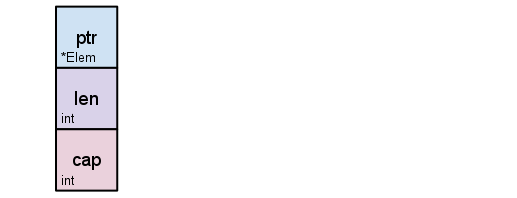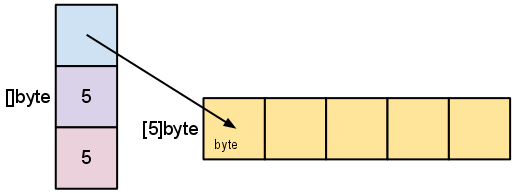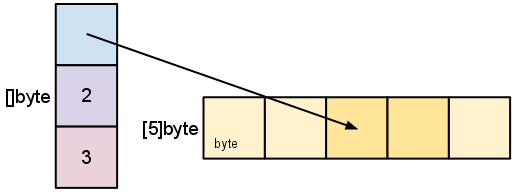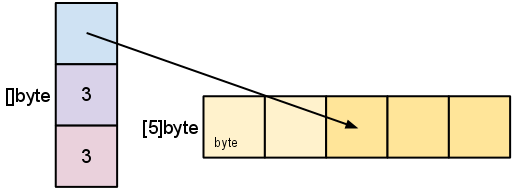# Go Slices: usage and internals

## Introduction

Go’s slice type provides a convenient and efficient means of working with sequences of typed data. Slices are analogous to arrays in other languages, but have some unusual properties. This article will look at what slices are and how they are used.

## Arrays

The slice type is an abstraction built on top of Go’s array type, and so to understand slices we must first understand arrays.

An array type definition specifies a length and an element type. For example, the type `int` represents an array of four integers. An array’s size is fixed; its length is part of its type (`int` and `int` are distinct, incompatible types). Arrays can be indexed in the usual way, so the expression `s[n]` accesses the nth element, starting from zero.

``````var a int
a = 1
i := a
// i == 1
``````

Arrays do not need to be initialized explicitly; the zero value of an array is a ready-to-use array whose elements are themselves zeroed:

``````// a == 0, the zero value of the int type
``````

The in-memory representation of `int` is just four integer values laid out sequentially:Go’s arrays are values. An array variable denotes the entire array; it is not a pointer to the first array element (as would be the case in C). This means that when you assign or pass around an array value you will make a copy of its contents. (To avoid the copy you could pass a pointer to the array, but then that’s a pointer to an array, not an array.) One way to think about arrays is as a sort of struct but with indexed rather than named fields: a fixed-size composite value.

An array literal can be specified like so:

``````b := string{"Penn", "Teller"}
``````

Or, you can have the compiler count the array elements for you:

``````b := [...]string{"Penn", "Teller"}
``````

In both cases, the type of `b` is `string`.

## Slices

Arrays have their place, but they’re a bit inflexible, so you don’t see them too often in Go code. Slices, though, are everywhere. They build on arrays to provide great power and convenience.

The type specification for a slice is `[]T`, where `T` is the type of the elements of the slice. Unlike an array type, a slice type has no specified length.

A slice literal is declared just like an array literal, except you leave out the element count:

``````letters := []string{"a", "b", "c", "d"}
``````

A slice can be created with the built-in function called `make`, which has the signature,

``````func make([]T, len, cap) []T
``````

where T stands for the element type of the slice to be created. The `make` function takes a type, a length, and an optional capacity. When called, `make` allocates an array and returns a slice that refers to that array.

``````var s []byte
s = make([]byte, 5, 5)
// s == []byte{0, 0, 0, 0, 0}
``````

When the capacity argument is omitted, it defaults to the specified length. Here’s a more succinct version of the same code:

``````s := make([]byte, 5)
``````

The length and capacity of a slice can be inspected using the built-in `len` and `cap` functions.

``````len(s) == 5
cap(s) == 5
``````

The next two sections discuss the relationship between length and capacity.

The zero value of a slice is `nil`. The `len` and `cap` functions will both return 0 for a nil slice.

A slice can also be formed by “slicing” an existing slice or array. Slicing is done by specifying a half-open range with two indices separated by a colon. For example, the expression `b[1:4]` creates a slice including elements 1 through 3 of `b` (the indices of the resulting slice will be 0 through 2).

``````b := []byte{'g', 'o', 'l', 'a', 'n', 'g'}
// b[1:4] == []byte{'o', 'l', 'a'}, sharing the same storage as b
``````

The start and end indices of a slice expression are optional; they default to zero and the slice’s length respectively:

``````// b[:2] == []byte{'g', 'o'}
// b[2:] == []byte{'l', 'a', 'n', 'g'}
// b[:] == b
``````

This is also the syntax to create a slice given an array:

``````x := string{"Лайка", "Белка", "Стрелка"}
s := x[:] // a slice referencing the storage of x
``````

## Slice internals

A slice is a descriptor of an array segment. It consists of a pointer to the array, the length of the segment, and its capacity (the maximum length of the segment).Our variable `s`, created earlier by `make([]byte, 5)`, is structured like this:The length is the number of elements referred to by the slice. The capacity is the number of elements in the underlying array (beginning at the element referred to by the slice pointer). The distinction between length and capacity will be made clear as we walk through the next few examples.

As we slice `s`, observe the changes in the slice data structure and their relation to the underlying array:

``````s = s[2:4]
``````Slicing does not copy the slice’s data. It creates a new slice value that points to the original array. This makes slice operations as efficient as manipulating array indices. Therefore, modifying the elements (not the slice itself) of a re-slice modifies the elements of the original slice:

``````d := []byte{'r', 'o', 'a', 'd'}
e := d[2:]
// e == []byte{'a', 'd'}
e = 'm'
// e == []byte{'a', 'm'}
// d == []byte{'r', 'o', 'a', 'm'}
``````

Earlier we sliced `s` to a length shorter than its capacity. We can grow s to its capacity by slicing it again:

``````s = s[:cap(s)]
``````A slice cannot be grown beyond its capacity. Attempting to do so will cause a runtime panic, just as when indexing outside the bounds of a slice or array. Similarly, slices cannot be re-sliced below zero to access earlier elements in the array.

## Growing slices (the copy and append functions)

To increase the capacity of a slice one must create a new, larger slice and copy the contents of the original slice into it. This technique is how dynamic array implementations from other languages work behind the scenes. The next example doubles the capacity of `s` by making a new slice, `t`, copying the contents of `s` into `t`, and then assigning the slice value `t` to `s`:

``````t := make([]byte, len(s), (cap(s)+1)*2) // +1 in case cap(s) == 0
for i := range s {
t[i] = s[i]
}
s = t
``````

The looping piece of this common operation is made easier by the built-in copy function. As the name suggests, copy copies data from a source slice to a destination slice. It returns the number of elements copied.

``````func copy(dst, src []T) int
``````

The `copy` function supports copying between slices of different lengths (it will copy only up to the smaller number of elements). In addition, `copy` can handle source and destination slices that share the same underlying array, handling overlapping slices correctly.

Using `copy`, we can simplify the code snippet above:

``````t := make([]byte, len(s), (cap(s)+1)*2)
copy(t, s)
s = t
``````

A common operation is to append data to the end of a slice. This function appends byte elements to a slice of bytes, growing the slice if necessary, and returns the updated slice value:

``````func AppendByte(slice []byte, data ...byte) []byte {
m := len(slice)
n := m + len(data)
if n > cap(slice) { // if necessary, reallocate
// allocate double what's needed, for future growth.
newSlice := make([]byte, (n+1)*2)
copy(newSlice, slice)
slice = newSlice
}
slice = slice[0:n]
copy(slice[m:n], data)
return slice
}
``````

One could use `AppendByte` like this:

``````p := []byte{2, 3, 5}
p = AppendByte(p, 7, 11, 13)
// p == []byte{2, 3, 5, 7, 11, 13}
``````

Functions like `AppendByte` are useful because they offer complete control over the way the slice is grown. Depending on the characteristics of the program, it may be desirable to allocate in smaller or larger chunks, or to put a ceiling on the size of a reallocation.

But most programs don’t need complete control, so Go provides a built-in `append` function that’s good for most purposes; it has the signature

``````func append(s []T, x ...T) []T
``````

The `append` function appends the elements `x` to the end of the slice `s`, and grows the slice if a greater capacity is needed.

``````a := make([]int, 1)
// a == []int{0}
a = append(a, 1, 2, 3)
// a == []int{0, 1, 2, 3}
``````

To append one slice to another, use `...` to expand the second argument to a list of arguments.

``````a := []string{"John", "Paul"}
b := []string{"George", "Ringo", "Pete"}
a = append(a, b...) // equivalent to "append(a, b, b, b)"
// a == []string{"John", "Paul", "George", "Ringo", "Pete"}
``````

Since the zero value of a slice (`nil`) acts like a zero-length slice, you can declare a slice variable and then append to it in a loop:

``````// Filter returns a new slice holding only
// the elements of s that satisfy fn()
func Filter(s []int, fn func(int) bool) []int {
var p []int // == nil
for _, v := range s {
if fn(v) {
p = append(p, v)
}
}
return p
}
``````

## A possible “gotcha”

As mentioned earlier, re-slicing a slice doesn’t make a copy of the underlying array. The full array will be kept in memory until it is no longer referenced. Occasionally this can cause the program to hold all the data in memory when only a small piece of it is needed.

For example, this `FindDigits` function loads a file into memory and searches it for the first group of consecutive numeric digits, returning them as a new slice.

``````var digitRegexp = regexp.MustCompile("[0-9]+")

func FindDigits(filename string) []byte {
return digitRegexp.Find(b)
}
``````

This code behaves as advertised, but the returned `[]byte` points into an array containing the entire file. Since the slice references the original array, as long as the slice is kept around the garbage collector can’t release the array; the few useful bytes of the file keep the entire contents in memory.

To fix this problem one can copy the interesting data to a new slice before returning it:

``````func CopyDigits(filename string) []byte {
b = digitRegexp.Find(b)
c := make([]byte, len(b))
copy(c, b)
return c
}
``````

A more concise version of this function could be constructed by using `append`. This is left as an exercise for the reader.